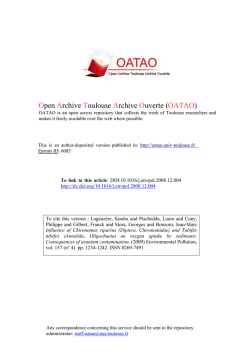# CUMULATIVE VOLUME OF WATER REQUIRED TO CARVE THE

```46th Lunar and Planetary Science Conference (2015)
1325.pdf
CUMULATIVE VOLUME OF WATER REQUIRED TO CARVE THE NOACHIAN VALLEY
NETWORKS. E. N. Rosenberg1 and J. W. Head1, 1Department of Earth, Environmental, and Planetary Sciences,
Brown University, Providence, RI 02912.
Introduction: The Martian valley networks, a majority of which ceased activity by the Noachian-Hesperian boundary , represent an excellent source of information about the climate of ancient Mars. Their formation required sustained flow of noncatastrophic
fluxes of liquid water, which would not be possible on
present-day Mars . However, there are several competing hypotheses as to the mechanism that supplied the
water [2-4]. Here, we first perform an order of magnitude estimate of the volume of water required to carve
the valleys, which puts a constraint on plausible hypotheses for the source of the water that eroded the valley
networks. Then we develop a method for estimating the
cumulative water volume required to erode a particular
valley network, which, when implemented, will place
further constraints on plausible formation hypotheses.
Methods: The required cumulative volume of water
depends on the strength of the flow through each valley
network. A very weak flow is highly inefficient at transporting sediment, so large quantities of water would be
required to produce the observed erosion. A strong flow
is more efficient, so less water would be required .
We define flow strength  as / , where  is the shear
stress between the bottom of the flow and the bed and
is the critical value of this parameter required to initiate transport of 50% of the sediment particles (those
with diameters smaller than the median diameter 50 ).
is equivalent to  ∗ ⁄∗ , where  ∗ is a dimensionless
ℎ
parameter called the Shields stress, which is equal to ,

where ℎ is the hydraulic radius (or approximately the
flow depth when the channel width ≫ depth),  is the
slope,  is a representative grain size (here 50 ), and
is the submerged specific gravity of the sediment (equal
to  / − 1, where  and  are, respectively, the densities of sediment and water). To estimate ∗ , we use
Equation 2-59a from :
−0.6
−0.6
∗ = 0.22
+ 0.06 exp(−17.77
),
where  ≔ √/, in which  is the acceleration
due to gravity and  is the kinematic viscosity of water.
We take this equation to account for all of the effects of
gravity. To see this, note that the fluid flux is given by
= ℎ = √8⁄ √ℎℎ,
where  is the mean flow speed,  is the flow width, ℎ
is the mean flow depth, and  is the Darcy-Weisbach
friction factor, which is a function of  and ℎ (see, for
example, Equations 2-29 and 2-30 of ). Similarly, the
bedload sediment flux is given by
=  √3 ,
where  , called the Einstein parameter, is a function of
∗ and ∗ (see Equation 2-89 of  and the subsequent
examples). Thus, when  and  are divided, the √
cancels, and the only dependence on  is through ∗ .
This dependence is very weak. For reasonable values of
R, D, and , ∗ only decreases by at most about 10% in
going from Earth gravity to Mars gravity. In our analysis here, we include the suspended sediment, in addition
to the bedload sediment, but we assume that the dependence on  is still primarily through ∗ . Note also that,
using many of the equations for  given in , the ratio
/ is primarily a function of . Thus, we use this as
our measure of flow strength.
From measurements of terrestrial rivers reported in
the literature , we find an empirical relationship between the ratio (volumetric fluid flux)/(volumetric sediment flux) =  / and the flow strength . We then
multiply this ratio by an estimate of the total eroded volume of the valley networks in order to estimate the volume of water that would be required for different flow
strengths.
Fluid/Sediment Flux Ratio: By plotting the data
compiled by  (after converting the sediment fluxes to
volumetric fluxes rather than mass fluxes and eliminating data points which infinite values for the ratio), we
obtain the following empirical relationship between
and  / :

−0.1778
=  11.95
.

As seen in Figure 1, the predicted ratios are accurate to
about an order of magnitude in either direction.
Figure 1: Empirical relationship between  / and
(top) and histogram showing error of regression curve
(bottom).
46th Lunar and Planetary Science Conference (2015)
Thus, for  = 3, we predict a fluid/sediment flux ratio
on the order of 10,000 (or the range 1,000 –100,000
within error). Similarly, for  = 10, we predict a ratio
on the order of 1000, and for  = 100, we predict a ratio
on the order of 100. For comparison, in the Mississippi
River,  varies between ~2 at the shallowest point (1 m
deep at headwaters) and ~120 at the deepest point (60 m
deep at New Orleans) (calculated using data from ). Similarly, for the Colorado River,  varies between
~130 at the shallowest point (2 m) and ~1000 at the
deepest point (30 m) (calculated using data from ).
Water Volume: Based on data provided by Williams and Phillips [11-12] and Hoke, et al. , we estimate the total volume of sediment eroded out of the
valley networks to be at least, and on the order of,
104 km3 . To calculate the cumulative volume of water
required to produce this erosion requires knowledge of
time-varying fluid fluxes and flow strengths in each of
the valleys. However, to make an order of magnitude
estimate, we assume a representative value of  to be
between 3 and 100. Hence, we favor cumulative water
volumes on the order of 106 − 108 km3 , corresponding
to global equivalent layers on the order of 10 m – 1 km.
With the error on the empirical fit, this interval becomes
1 m – 10 km. Note that this volume of water need not
have existed on Mars at any one time; it represents the
cumulative volume, including recycled water. For comparison, using the sediment volumes and estimated fluid
and sediment fluxes from  leads to a required water
volume of ~10 km GEL. However, the methods used in
 to estimate the flow depth rely on terrestrial analogies and assumptions about stability rather than estimates of the instantaneous fluid flux into the river,
which is what physically determines the flow depth. We
suggest that using the latter would be a more reliable
method.
We note that if the erosion was into bedrock, more
water would have been required, as the flow must also
do work to break the sediment particles loose .
Next Steps: The globally representative flow
strength  is poorly constrained. Thus, a better approach
would be to estimate the volume of water required to
erode particular valley networks. This is a function of
(1) the geometry of the valley, (2) the sediment grain
size and density, and (3) the hypothetical volumetric
fluid flux into the valley, which together determine the
flow depth h and flow strength . Thus, they determine,
to an order of magnitude, the water volume required to
erode the valley. (1) can be measured from stereo-image
DEMs and MOLA data [11-13]. (2) can be estimated
from what is known of the minerology and geologic history of Mars and from lander/rover data. (3) is dependent on the hypothetical source of the water that carved
1325.pdf
the valleys. Thus, this method can be used to check the
consistency of the hypotheses in question, such as rainfall in a warm and wet climate , meltwater in a lateNoachian icy highlands model , or rainfall induced
by giant impacts . For example, if a hypothesis predicts a low instantaneous fluid flux, then large cumulative fluid volumes would be required. But if these volumes are too large to have been provided by the hypothetical mechanism in a reasonable amount of time, then
this is reason to reject the hypothesis.
References:  C. Fassett and J. W. Head, Icarus,
195, 61 (2008).  R. Craddock and A. Howard, J. Geophys. Res., 107, no. E11, 5111 (2002).  I. Halevy
and J. W. Head, Nature Geoscience, 7, 865 (2014). 
T. Segura, et al., J. Geophys. Res, 113, E1107 (2008).
 M. Garcia, "Sediment Transport and Morphodynamics," in Sedimentation Engineering: Processes, Measurements, Modeling, and Practice, Reston, Virginia,
American Society of Civil Engineers (ASCE) (2008),
21-163.  T. Cleveland, et al., "Technical Memorandum 0-6549: Hydraulic Performance of Staggered-Barrel Culverts for Stream Crossings: Literature Review,"
Texas Tech University (2010).  L. Moya and G. Haglund, "Mississippi River Resource Page," Caleuche
(2014). [Online]. [Accessed 2014].  C. F. Nordin and
B. S. Queen, "Particle Size Distributions of Bed Sediments Along the Thalweg of the Mississippi River,
Cairo, Illinois, to Head of Passes, September 1989," US
Army Corps of Engineers: Lower Mississippi Valley
Division, Vicksburg, MS, 1992.  B. Ribokas, "Grand
Canyon Explorer: The Colorado River" (2000).
[Online]. [Accessed 2014].  D. M. Rubin and e. al.,
"20,000 Grain Size Observations from the Bed of the
Colorado River, and Implications for Sediment
Transport Through the Grand Canyon," in 2nd Joint
Federal Interagency Conference on Sedimentation and
Hydrologic Modeling, Las Vegas, Nevada (2010). 
R. Williams and R. Phillips, J. Geophys. Res, 106,
23737 (2001).  R. Williams, personal communication (2014).  M. Hoke and et al., Earth and Planetary Science Letters, 312, 1 (2011).  M. Lamb, et al.,
J. Geophys. Res, 113, F03025 (2008).  T. Segura, B.
Toon and A. Colaprete, J. Geophys. Res, 113, E1107,
(2008).
Acknowledgements: ENR received support from a
Brown University Undergraduate Teaching and Research
Award (UTRA). ENR and JWH are currently supported by a
Mars Express/NASA Project Extended Mission grant. This
```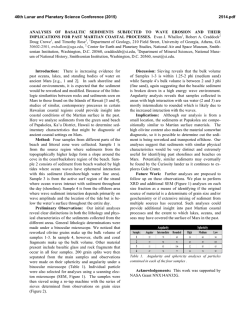# Analyses of Basaltic Sediments Subjected to Wave Erosion and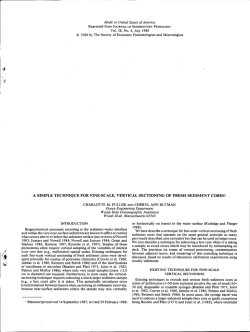# a simple technique for fine-scale, vertical sectioning of fresh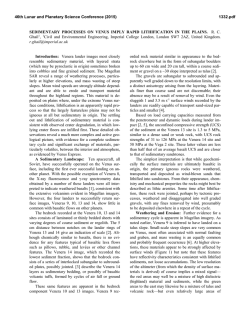# Sedimentary Processes on Venus Imply Rapid Lithification in the# Using the Al-sense panty liner to detect amniotic fluid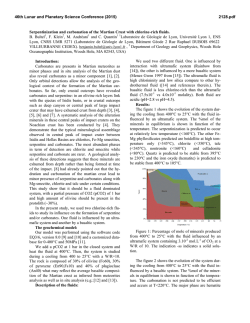# Serpentinization and carbonation of the Martian Crust with chlorine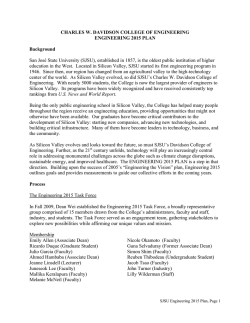# (SJSU), established in 18 - Charles W. Davidson College of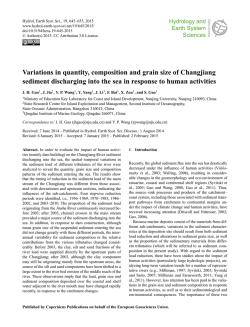# Variations in quantity, composition and grain size of Changjiang